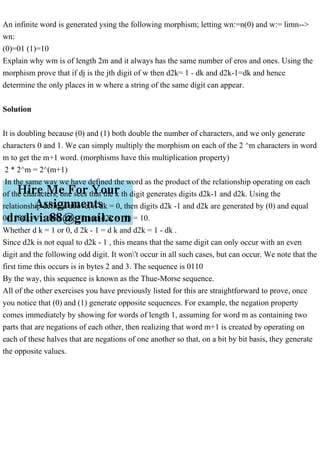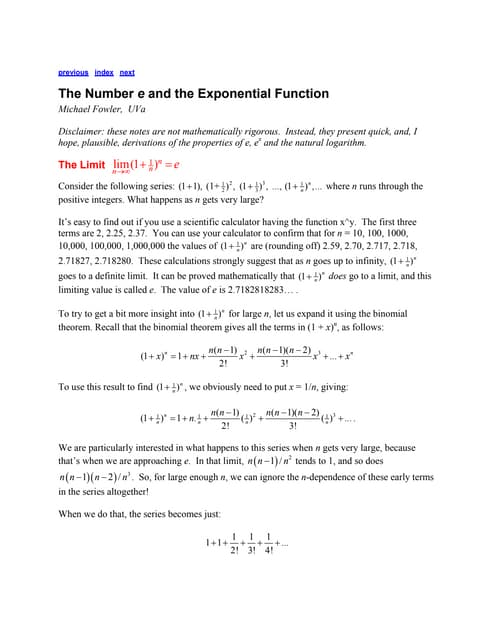PublicitéProchain SlideShareExponential function
Chargement dans ... 3
1 sur 1
Publicité

### An infinite word is generated ysing the following morphism; letting .pdf

1. An infinite word is generated ysing the following morphism; letting wn:=n(0) and w:= limn--> wn: (0)=01 (1)=10 Explain why wm is of length 2m and it always has the same number of eros and ones. Using the morphism prove that if dj is the jth digit of w then d2k= 1 - dk and d2k-1=dk and hence determine the only places in w where a string of the same digit can appear. Solution It is doubling because (0) and (1) both double the number of characters, and we only generate characters 0 and 1. We can simply multiply the morphism on each of the 2 ^m characters in word m to get the m+1 word. (morphisms have this multiplication property) 2 * 2^m = 2^(m+1) In the same way we have defined the word as the product of the relationship operating on each of the characters, one sees that the k th digit generates digits d2k-1 and d2k. Using the relationship defined above, if dk = 0, then digits d2k -1 and d2k are generated by (0) and equal 01; if dk = 1, then d2k - 1 and d2k = (1) = 10. Whether d k = 1 or 0, d 2k - 1 = d k and d2k = 1 - dk . Since d2k is not equal to d2k - 1 , this means that the same digit can only occur with an even digit and the following odd digit. It won't occur in all such cases, but can occur. We note that the first time this occurs is in bytes 2 and 3. The sequence is 0110 By the way, this sequence is known as the Thue-Morse sequence. All of the other exercises you have previously listed for this are straightforward to prove, once you notice that (0) and (1) generate opposite sequences. For example, the negation property comes immediately by showing for words of length 1, assuming for word m as containing two parts that are negations of each other, then realizing that word m+1 is created by operating on each of these halves that are negations of one another so that, on a bit by bit basis, they generate the opposite values.
Publicité